# How to answer unit conversion questions on the SAT Math sectionThe SAT Math section requires a comprehensive understanding of proportions, rates, and ratios. These concepts are often tested through unit conversion questions, which give you a measurement (height, weight, time, distance, etc.) and then ask you to convert that measurement into a different unit. For example, a question might tell you how many pounds a backpack is and then ask you to calculate its weight in ounces. Or it might give you a student’s height in feet and then ask for her height in inches.

Students who struggle with these questions generally have trouble setting up the problems. The good news is that once you’ve written down all the important information and set up the correct ratio, the rest is simple arithmetic. Let’s take a look at a process you can use to set up and calculate unit conversion questions.

#### Step 1: Identify what you need.

Example: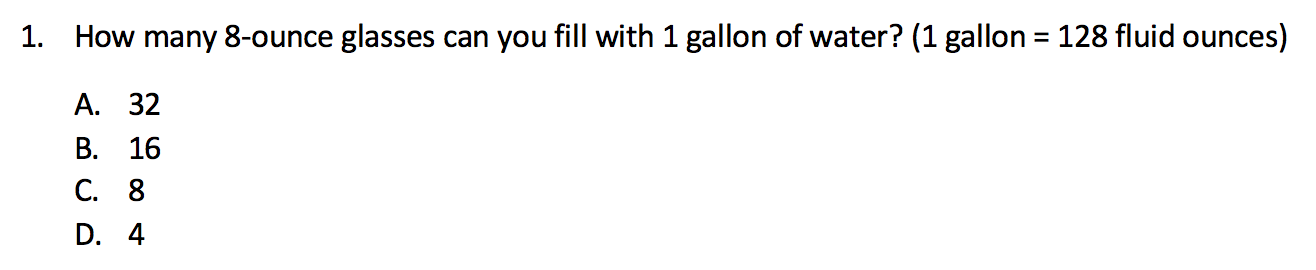We need to find the number of glasses. There are two units of measurement given in the question: gallons and ounces. Glasses are given in terms of ounces, so we must convert from gallons to ounces.

#### Step 2: Set up your first proportion.

Write a proportion that shows the relationship between your two given units of measurement. If you’re uncertain where to find that information, try looking at the end of the question in the parentheses.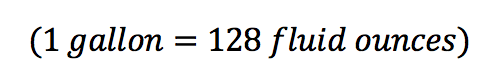We know that for every 1 gallon, there are 128 ounces, so we can write the ratio like this: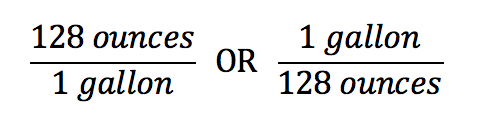These are the same. Choose whichever you find most useful.

#### Step 3: Build your chain.

Once you have your first proportion written down, it’s time to think about your goal. We need to find the number of glasses. Since glasses are given in terms of ounces, our next proportion should show the relationship between glasses and ounces. The question tells us 1 glass has 8 ounces, so we can write the proportion like this: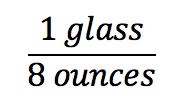Multiply your two proportions together to solve for glasses.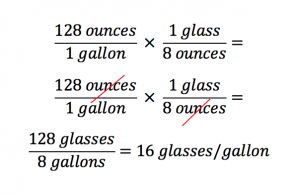There are 16 glasses for every gallon.

Notice how the ounces cancel to leave you with glasses per gallon. If you set up your proportions and solve correctly, only your desired units will remain.

#### Example

Let’s try another.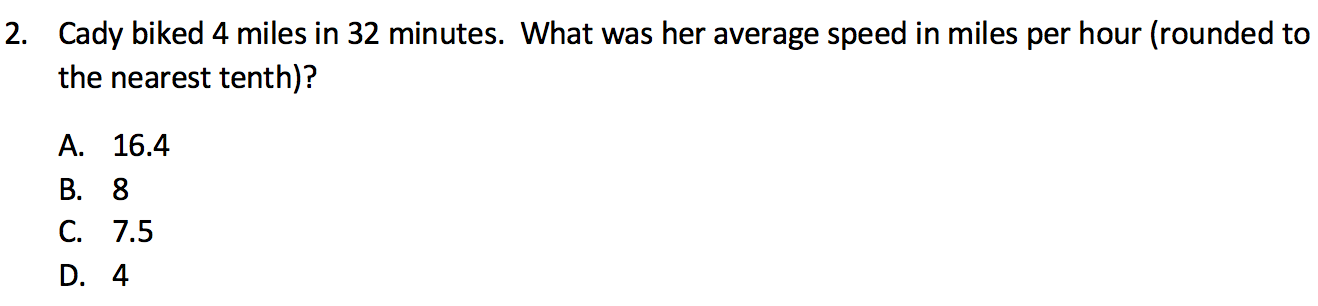This question asks us to find Cady’s “average speed in miles per hour,” so we know our answer choices are in miles per hour. Our starting proportion may be trickier to locate. Because there’s no parenthetical conversion in this problem, we need to infer which conversion to use. We’re given minutes, but we need to convert to hours, so there is an implied conversion: 1 hour = 60 minutes.

Since we're given minutes but need to find hours, we’ll start with minutes.

Let’s make our first proportion by putting the given number of minutes on top and the corresponding number of miles on bottom.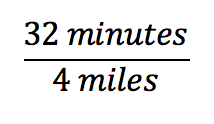Next, we’ll make our chain. We want minutes to cancel and hours to remain, so we’ll put minutes on the bottom and hours on top. In this case, it makes sense to put everything in terms of 1 hour.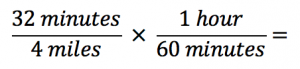Finally, let’s multiply across to calculate our remaining value.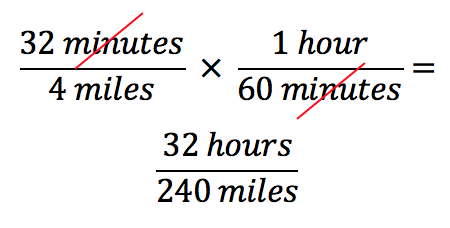Before going any further, notice we’ve reversed our desired units. Though we’re looking for miles per hour, we’ve arrived at hours per mile. Never fear! We need only flip the proportion before continuing on our journey.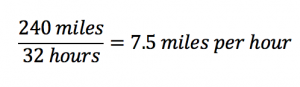Mastering the proportion chain can be a powerful tool going into the SAT Math section. While unit conversion questions are certainly the most common way that the SAT will test your understanding of measurements and their conversions, any SAT Math question could try to trick you by beginning a question with one unit of measurement and then switching the language to refer to another unit of measurement in the answer choices. Remain ever vigilant when noting units and always be ready to convert as needed!

For more tips, download our guide to the 6 skills you need to master for the SAT.

The recommendations above are general suggestions. If you have specific questions about your individual test prep plans, reach out to our experts here. We’re happy to help in any way we can.

##### Related Posts